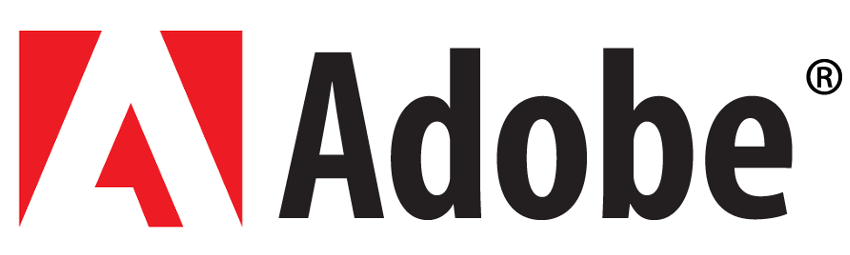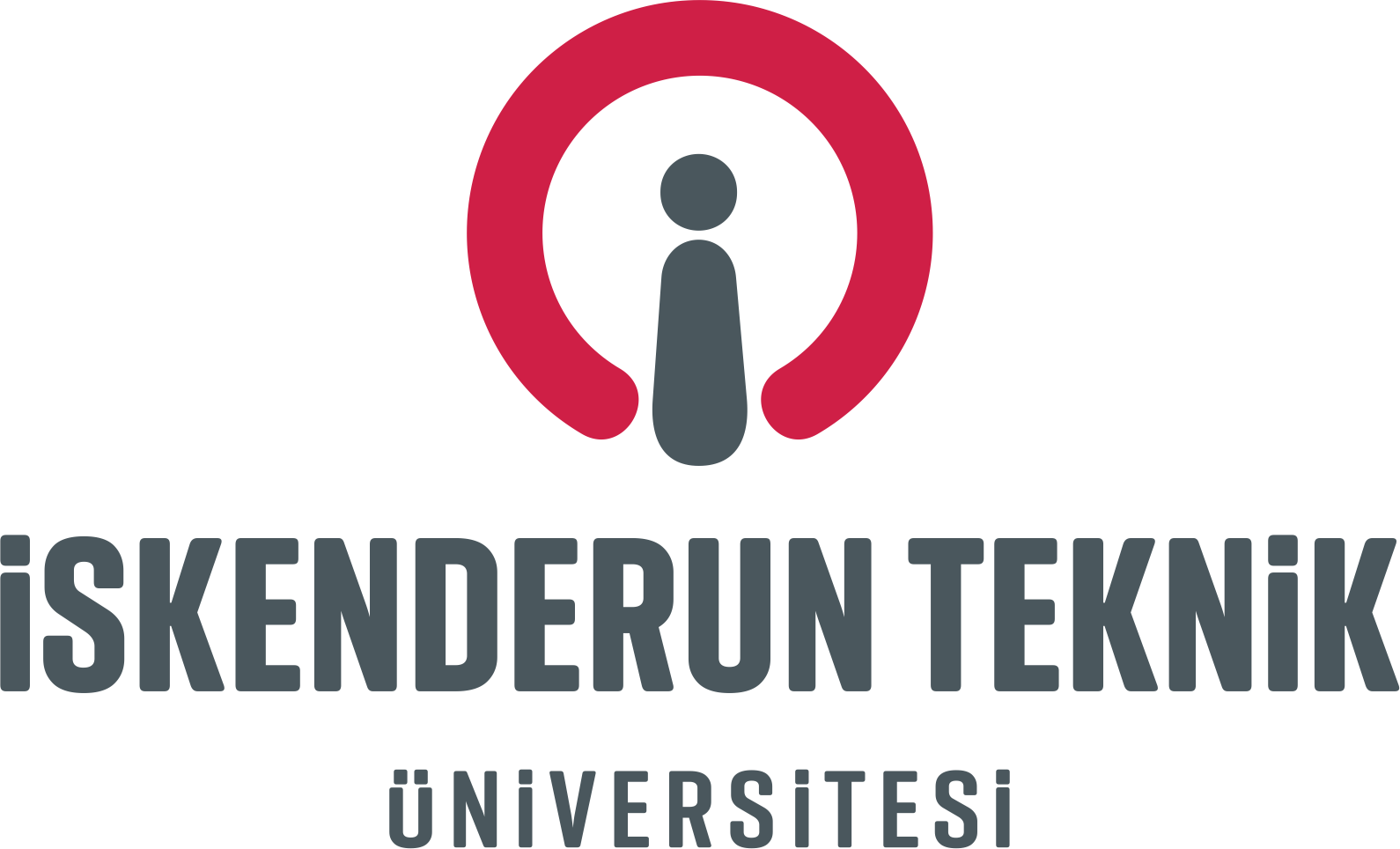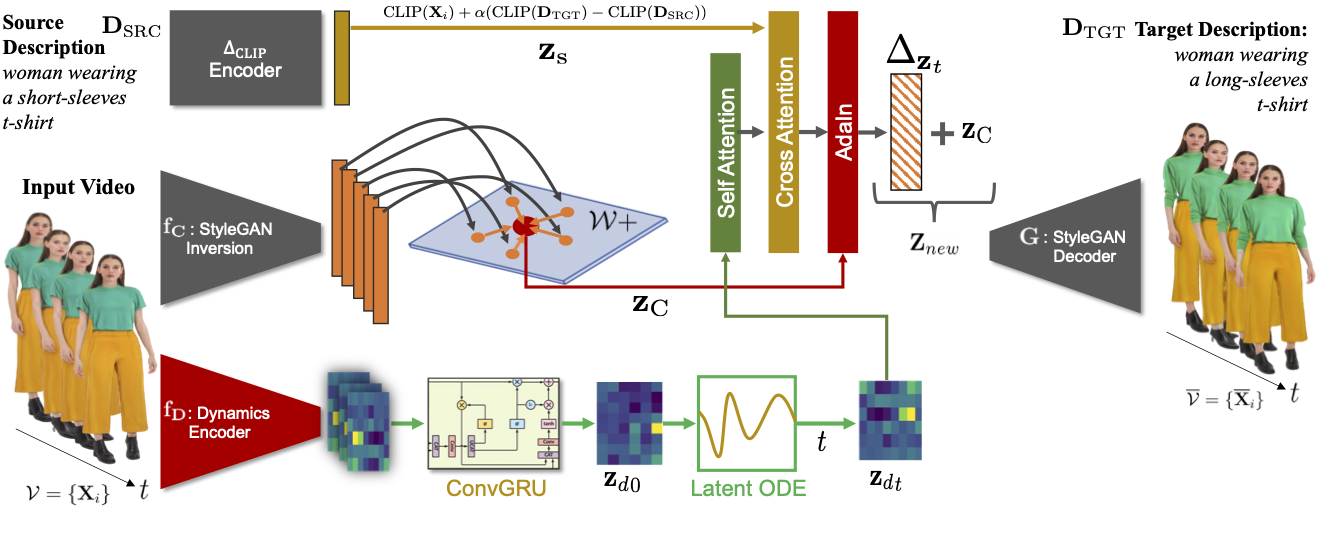# VidStyleODE

## Disentangled Video Editing via StyleGAN and NeuralODEs## Abstract

We propose VidStyleODE, a spatiotemporally continuous disentangled Video representation based upon StyleGAN and Neural-ODEs. Effective traversal of the latent space learned by Generative Adversarial Networks (GANs) has been the basis for recent breakthroughs in image editing. However, the applicability of such advancements to the video domain has been hindered by the difficulty of representing and controlling videos in the latent space of GANs. In particular, videos are composed of content (i.e., appearance) and complex motion components that require a special mechanism to disentangle and control. To achieve this, VidStyleODE encodes the video content in a pre-trained StyleGAN $$\mathcal{W}_+$$ space and benefits from a latent ODE component to summarize the spatiotemporal dynamics of the input video. Our novel continuous video generation process then combines the two to generate high-quality and temporally consistent videos with varying frame rates. We show that our proposed method enables a variety of applications on real videos: text-guided appearance manipulation, motion manipulation, image animation, and video interpolation and extrapolation.

 Paper Arxiv Code

## Method OverviewWe encode video dynamics and process them using a ConvGRU layer to obtain a dynamic latent representation $$\mathbf{Z}_{d0}$$ used to initialize a latent ODE of the motion (bottom). We also encode the video in $$\mathcal{W}_+$$ space to obtain a global latent code $$\mathbf{Z}_C$$ (middle). We combine the two with an external style cue through an attention mechanism to condition the AdaIN layer that predicts the directions to the latent codes of the frames in the target video (top). Modules in $$\textcolor{gray}{\textbf{gray}}$$ are $$\it{\textit{pre-trained}}$$ and $$\it{frozen}$$ during training.

## 1. Image Animation

We obtain the video global code from the given single frame and use the motion from another driving video by extracting its dynamic latent representation.

## 2. Text-Guided Appearance Manipulation

We manipulate a source video by specifying a direction based on a given source text that describes the video and alternative target descriptions.

## 3. Local Dynamic Editing

The rich dynamic representation learned by VidStyleODE, we are able to manipulate the motion of local body parts of a target video by blending its dynamic representation with another video.   $\mathbf{z}_{d_{new}} = m * \mathbf{z}_{d_1} + (1 - m) * \mathbf{z}_{d_2}$ $m \in \{0, 1\} ^ {\{ 8 \times 6\}}$

## 4. Temporal Interpolation and Extrapolation

We extract the dynamic representation from $$K$$ given frames of timestamps $$[t_1, t_2, \dots, t_k ]$$ and perform interpolation or extrapolation by solving the latent ODE in the intended timestamps.

## 5. Video Dynamic Latent Interpolation

To show the expressiveness of our learned motion representation, we interpolate between two different dynamic representations by taking a weighted average of the two and generating a new video accordingly.  $\mathbf{z}_{d_{new}} = (1-\lambda) * \mathbf{z}_{d_1} + \lambda * \mathbf{z}_{d_2}$

## 6. Ablation Study On Model Architecture

To demonstrate the effectiveness of the loss and architecture choices of VidStyleODE, we report sample generated videos of our method without its most essential components: consistency loss $$\mathcal{L}_C$$, structure loss $$\mathcal{L}_S$$, appearance loss $$\mathcal{L}_A$$, latent directions, and the conditional modulation network.

## 7. Failure Cases

BibTeX
 @misc{vidstyleode,
title={VidStyleODE: Disentangled Video Editing via StyleGAN and NeuralODEs},
author={Moayed Haji Ali and Andrew Bond and Tolga Birdal and Duygu Ceylan and Levent Karacan and Erkut Erdem and Aykut Erdem},
year={2023},
eprint={2304.06020},
archivePrefix={arXiv},
primaryClass={cs.CV}}

Contact